National Institute of Electronics & Information Technology (NIELIT) Scientist syllabus for electronics

Every year Nielit recruits scientist-B from various branches here i am giving syllabus for electronics and communications branch.

Electronics and Communication Engineering (EC):
Engineering Mathematics

Linear Algebra: Matrix Algebra, Systems of linear equations, Eigen values and eigen vectors.
Calculus: Mean value theorems, Theorems of integral calculus, Evaluation of definite and improper integrals, Partial Derivatives, Maxima and minima, Multiple integrals, Fourier series. Vector identities, Directional derivatives, Line, Surface and Volume integrals, Stokes, Gauss and Green’s theorems.
Differential equations: First order equation (linear and nonlinear), Higher order linear
differential equations with constant coefficients, Method of variation of parameters, Cauchy’s and Euler’s equations, Initial and boundary value problems, Partial Differential Equations and variable separable method.
Complex variables: Analytic functions, Cauchy’s integral theorem and integral formula,Taylor’s and Laurent’ series, Residue theorem, solution integrals.
Probability and Statistics: Sampling theorems, Conditional probability, Mean, median, mode and standard deviation, Random variables, Discrete and continuous distributions, Poisson,Normal and Binomial distribution, Correlation and regression analysis.
Numerical Methods: Solutions of non-linear algebraic equations, single and multi-step methods for differential equations.
Transform Theory: Fourier transform,Laplace transform, Z-transform.

Electronics and Communication Engineering technical

Networks:
Network graphs: matrices associated with graphs; incidence, fundamental cut set and fundamental circuit matrices. Solution methods: nodal and mesh analysis. Network theorems:
superposition, Thevenin and Norton’s maximum power transfer, Wye-Delta transformation.Steady state sinusoidal analysis using phasors. Linear constant coefficient differential equations;time domain analysis of simple RLC circuits, Solution of network equations using Laplacetransform: frequency domain analysis of RLC circuits. 2-port network parameters: driving pointand transfer functions. State equations for networks.
Electronic Devices: Energy bands in silicon, intrinsic and extrinsic silicon. Carrier transport in silicon: diffusion current, drift current, mobility, and resistivity. Generation and recombination of carriers.p-n junction diode, Zener diode, tunnel diode, BJT, JFET, MOS capacitor, MOSFET, LED, p-I-n and avalanche photo diode, Basics of LASERs. Device technology: integrated circuits fabrication process, oxidation, diffusion, ion implantation, photolithography, n-tub, p-tub and twin-tub CMOS process.
Analog Circuits: Small Signal Equivalent circuits of diodes, BJTs, MOSFETs and analog CMOS. Simple diode circuits, clipping, clamping, rectifier.Biasing and bias stability of transistor and FET amplifiers. Amplifiers: single-and multi-stage, differential and operational, feedback, and power. Frequency response of amplifiers.Simple op-amp circuits. Filters. Sinusoidal oscillators; criterion for oscillation; single-transistor and op-amp configurations.Function generators and wave-shaping circuits, 555 Timers. Power supplies.
Digital circuits: Boolean algebra, minimization of Boolean functions; logic gates; digital IC families (DTL, TTL, ECL, MOS, CMOS). Combinatorial circuits: arithmetic circuits, code converters, multiplexers, decoders, PROMs and PLAs. Sequential circuits: latches and flip-flops, counters and shift-registers. Sample and hold circuits, ADCs, DACs. Semiconductor memories.
Microprocessor(8085): architecture, programming, memory and I/O interfacing.
Signals and Systems: Definitions and properties ofLaplace transform, continuous-time and discrete-time Fourier series, continuous-time and discrete-time Fourier Transform, DFT and FFT, z-transform. Sampling theorem. Linear Time-Invariant (LTI) Systems: definitions and properties; causality, stability, impulse response, convolution, poles and zeros, parallel and cascade structure, frequency response, group delay, phase delay. Signal transmission through LTI systems.
Control Systems: Basic control system components; block diagrammatic description, reduction of block diagrams. Open loop and closed loop (feedback) systems and stability analysis of these systems. Signal flow graphs and their use in determining transfer functions of systems; transient and steady state analysis of LTI control systems and frequency response. Tools and techniques for LTI control system analysis: root loci, Routh-Hurwitz criterion, Bode and Nyquist plots.
Control system compensators: elements of lead and lag compensation, elements of Proportional- Integral-Derivative (PID) control. State variable representation and solution of state equation of LTI control systems.
Communications: Random signals and noise: probability, random variables, probability density function, autocorrelation, power spectral density. Analog communication systems: amplitude and angle modulation and demodulation systems, spectral analysis of these operations, superheterodyne receivers; elements of hardware, realizations of analog communication systems; signal-to-noise ratio (SNR) calculations for amplitude modulation (AM) and frequency modulation (FM) for low noise conditions. Fundamentals of information theory and channel capacity theorem. Digital communication systems: pulse code modulation (PCM), differential
pulse code modulation (DPCM), digital modulation schemes: amplitude, phase and frequency shift keying schemes (ASK, PSK, FSK), matched filter receivers, bandwidth consideration and probability of error calculations for these schemes. Basics of TDMA, FDMA and CDMA and GSM.
Electromagnetics: Elements of vector calculus: divergence and curl; Gauss’ and Stokes’ theorems, Maxwell’s equations: differential and integral forms. Wave equation, Poynting vector. Plane waves: propagation through various media; reflection and refraction; phase and group velocity; skin depth. Transmission lines: characteristic impedance; impedance transformation; Smith chart; impedance matching; S parameters, pulse excitation. Waveguides: modes in rectangular waveguides; boundary conditions; cut-off frequencies; dispersion relations. Basics of propagation in dielectric waveguide and optical fibers. Basics of Antennas: Dipole antennas;

Nielit scientist-B exam question paper pattern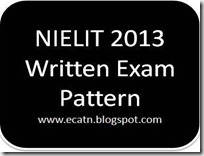National Institute of Electronics & Information Technology (NIELIT) recruits scientists from various branches every year, the selection process consists of written exam. The syllabus for this post was already given in my previous posts but here i am giving a flow chart of exam pattern which consists of both technical and non technical questions based on the syllabus.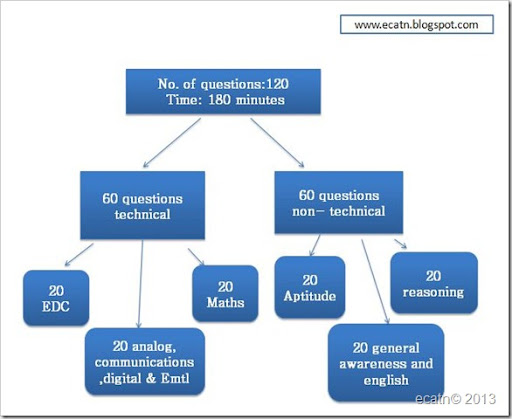Preparation Steps to Success in any competitive Exams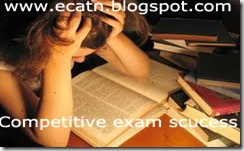Now a days there is a more competition for any competitive exams.Here I am giving some important steps based on my experience to success in any competitive exams related to electronics like BSNL, IES, GATE, BARC, DRDO, ISRO, BHEL, BEL, GAIL, etc……..

Step1: First step is read the notification completely and if they mention any exam date in notification note down the exam date,If they didn’t mention the date then just assume that the exam will be held with in 2 month from the release of notification(now note down the expected/assumed exam date).

Step2: Now next step is to know the syllabus and exam pattern ,search the internet/website of company and take the printout of the syllabus.

Step3: Most important step is to find previous year question papers,Search internet or Buy previous papers books in a market or online.The previous papers are used to give an idea about the exam pattern and to know what type of questions are giving.

Step4: Now check the previous papers questions, are these questions are coming from syllabus or not.If any question is from outside of the syllabus then you just add that topic into syllabus.

Step5: Until Step4 we collect the sources to prepare for the exam.Now according to the exam date and based on your convenience you should prepare a preparation daily plan/Time table.

Step6: According to the Plan/Time table Start your preparation.Before reading any text book first check the previous questions concert to that subject/topic and observe what type of questions are giving, and which areas they covered most,so that its becomes easy to study and give more interest to study.

Step7: Don’t spend more time on reading theory text books,Instead of that practice more objective questions of all previous papers of competitive exams that gives good confidence and shows the way to success..

ONE WHO PRACTICE A LOT WILL DEFINETLY GET SUCCESS.
ALL THE BEST!!!!

AP PGECET Syllabus and previous papers for ece and eee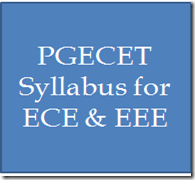The main objective of PGECET is to identify at the state level, meritorious and motivated candidates who didn’t qualify in GATE exam for admission to Post Graduate programmes in Engineering and technology. PGCET exam is useful for the students who wants to take admissions in higher education.  Here I am giving syllabus for APPGCET written exam for ECE and EEE.
PGECET examinations consist of each paper of 2 hours duration and carry a maximum of 120 marks.

Electronics & Communication Engineering:

Engineering Mathematics
Linear Algebra: Matrix Algebra, Systems of linear equations, Eigen values and Eigen vectors.
Calculus: Mean value theorems, Theorems of integral calculus, Evaluation of definite and improper integrals,Partial Derivatives, Maxima and minima, multiple integrals, Fourier series. Vector identities, Directional derivatives, Line, Surface and Volume integrals, Stokes, Gauss and Green's theorems.
Differential equations: First order equation (linear and nonlinear), Higher order linear differential equations with constant coefficients, Method of variation of parameters, Cauchy's and Euler's equations, Initial and boundary value problems, Partial Differential Equations and variable separable method.
Complex variables: Analytic functions, Cauchy's integral theorem and integral formula, Taylor's and Laurent' series, Residue theorem, solution integrals.
Probability and Statistics: Sampling theorems, Conditional probability, Mean, median, mode and standard deviation, Random variables, Discrete and continuous distributions, Poisson, Normal and Binomial distribution, Correlation and regression analysis.
Numerical Methods: Solutions of non-linear algebraic equations, single and multi-step methods for
differential equations.
Networks: Network graphs: matrices associated with graphs; incidence, fundamental cut set and fundamental circuit matrices. Solution methods: nodal and mesh analysis. Network theorems: superposition, Thevenin and Norton's maximum power transfer, Wye-Delta transformation. Steady state sinusoidal analysis using phasors. Linear constant coefficient differential equations; time domain analysis of simple RLC circuits, Solution of network equations using Laplace transform: frequency domain analysis of RLC circuits. 2-port network parameters: driving point and transfer functions. State equations for networks.
Electronic Devices: Energy bands in silicon, intrinsic and extrinsic silicon. Carrier transport in silicon:
diffusion current, drift current, mobility, and resistivity. Generation and recombination of carriers. p-n junction diode, Zener diode, tunnel diode, BJT, JFET, MOS capacitor, MOSFET, LED, p-I-n and avalanche photo diode, Basics of LASERs. Device technology: integrated circuits fabrication process, oxidation, diffusion, ion implantation, photolithography, n-tub, p-tub and twin-tub CMOS process.

Analog Circuits: Small Signal Equivalent circuits of diodes, BJTs, MOSFETs and analog CMOS. Simple diode circuits, clipping, clamping, rectifier. Biasing and bias stability of transistor and FET amplifiers.Amplifiers: single-and multi-stage, differential and operational, feedback, and power. Frequency response of amplifiers. Simple op-amp circuits. Filters. Sinusoidal oscillators; criterion for oscillation; single-transistor and op-amp configurations. Function generators and wave-shaping circuits, 555 Timers. Power supplies.
Digital circuits: Boolean algebra, minimization of Boolean functions; logic gates; digital IC families (DTL,TTL, ECL, MOS, CMOS). Combinatorial circuits: arithmetic circuits, code converters, multiplexers, decoders,PROMs and PLAs. Sequential circuits: latches and flip-flops, counters and shift-registers. Sample and hold circuits, ADCs, DACs. Semiconductor memories. Microprocessor (8085): architecture, programming, memory and I/O interfacing.
Signals and Systems: Definitions and properties of Laplace transform continuous-time and discrete-time Fourier series, continuous-time and discrete-time Fourier Transform, DFT and FFT, ztransform. Sampling theorem. Linear Time-Invariant (LTI) Systems: definitions and properties; causality, stability, impulse response, convolution, poles and zeros, parallel and cascade structure, frequency response, group delay, phase delay. Signal transmission through LTI systems.
Control Systems: Basic control system components; block diagrammatic description, reduction of block diagrams. Open loop and closed loop (feedback) systems and stability analysis of these systems. Signal flow graphs and their use in determining transfer functions of systems; transient and steady state analysis of LTI control systems and frequency response. Tools and techniques for LTI control system analysis: root loci, Routh-Hurwitz criterion, Bode and Nyquist plots. Control system compensators: elements of lead and lag compensation, elements of Proportional-Integral-Derivative (PID) control. State variable representation and solution of state equation of LTI control systems.
Communications: Random signals and noise: probability, random variables, probability density function, autocorrelation, power spectral density. Analog communication systems: amplitude and angle modulation and demodulation systems, spectral analysis of these operations, superheterodyne receivers; elements of hardware, realizations of analog communication systems; signal-to-noise ratio (SNR) calculations for amplitude modulation (AM) and frequency modulation (FM) for low noise conditions. Fundamentals of information theory and channel capacity theorem. Digital communication systems: pulse code modulation (PCM), differential pulse code modulation (DPCM), digital modulation schemes: amplitude, phase and frequency shift keying schemes (ASK, PSK, FSK), matched filter receivers, bandwidth consideration and probability of error calculations for these schemes. Basics of TDMA, FDMA and CDMA and GSM.
Electromagnetics: Elements of vector calculus: divergence and curl; Gauss' and Stokes' theorems, Maxwell's equations: differential and integral forms. Wave equation, Poynting vector. Plane waves: propagation through various media; reflection and refraction; phase and group velocity; skin depth. Transmission lines: characteristic impedance; impedance transformation; Smith chart; impedance matching; S parameters, pulse excitation. Waveguides: modes in rectangular waveguides; boundary conditions; cut-off frequencies;dispersion relations. Basics of propagation in dielectric waveguide and optical fibers. Basics of Antennas: Dipole antennas; radiation pattern; antenna gain.

Electrical Engineering:

Mathematics: Similar to ECE syllabus.
Electric Circuits and Fields: Network graph, KCL, KVL, node and mesh analysis, transient response of dc and ac networks; sinusoidal steady-state analysis, resonance, basic filter concepts; ideal current and voltage sources, Thevenin's, Norton's and Superposition and Maximum Power Transfer theorems, two-port networks, three phase circuits; Gauss Theorem, electric field and potential due to point, line, plane and spherical charge distributions; Ampere's and Biot-Savart's laws; inductance; dielectrics; capacitance.
Signals and Systems: Representation of continuous and discrete-time signals; shifting and scaling operations; linear, time-invariant and causal systems; Fourier series representation of continuous periodic signals; sampling theorem; Fourier, Laplace and Z transforms.
Electrical Machines: Single phase transformer - equivalent circuit, phasor diagram, tests, regulation and efficiency; three phase transformers - connections, parallel operation; auto-transformer; energy conversion principles; DC machines - types, windings, generator characteristics, armature reaction and commutation, starting and speed control of motors; three phase induction motors - principles, types, performance characteristics, starting and speed control; single phase induction motors; synchronous machines -performance, regulation and parallel operation of generators, motor starting, characteristics and applications;servo and stepper motors.
Power Systems: Basic power generation concepts; transmission line models and performance; cable
performance, insulation; corona and radio interference; distribution systems; per-unit quantities; bus
impedance and admittance matrices; load flow; voltage control; power factor correction; economic operation;symmetrical components; fault analysis; principles of over-current, differential and distance protection; solid state relays and digital protection; circuit breakers; system stability concepts, swing curves and equal area criterion; HVDC transmission and FACTS concepts.
Control Systems: Principles of feedback; transfer function; block diagrams; steady-state errors; Routh and Niquist techniques; Bode plots; root loci; lag, lead and lead-lag compensation; state space model; state transition matrix, controllability and observability.
Electrical and Electronic Measurements: Bridges and potentiometers; PMMC, moving iron, dynamometer and induction type instruments; measurement of voltage, current, power, energy and power factor; instrument transformers; digital voltmeters and multimeters; phase, time and frequency measurement; Q-meters; oscilloscopes; potentiometric recorders; error analysis.
Analog and Digital Electronics: Characteristics of diodes, BJT, FET; amplifiers - biasing, equivalent circuit and frequency response; oscillators and feedback amplifiers; operational amplifiers - characteristics and applications; simple active filters; VCOs and timers; combinational and sequential logic circuits; multiplexer; Schmitt trigger; multi-vibrators; sample and hold circuits; A/D and D/A converters; 8-bit microprocessor basics, architecture, programming and interfacing.
Power Electronics and Drives: Semiconductor power diodes, transistors, thyristors, triacs, GTOs, MOSFETs and IGBTs - static characteristics and principles of operation; triggering circuits; phase control rectifiers; bridge converters - fully controlled and half controlled; principles of choppers and inverters; basis concepts of adjustable speed dc and ac drives.

Best top GATE Coaching centers in India-Hyderabad-Chennai-DelhiAfter completion of my graduation I was thinking what to do next, at that time some one suggested me to take GATE exam for higher studies so,I decided to write GATE for that purpose I asked so many people who got success in previous GATE exams about the coaching centers i.e I almost done a small research on coaching centers. As per my survey most of the people suggest the below four coaching centers only so here I want to share my research with you.

1. ACE Academy: In every year thousands of people are taking coaching from this institute.This is the one of the best institute which succeeded in giving better coaching to GATE and it is located in Hyderabad.
2. Made Easy: This is also one of the successful institute for Both IES and GATE coaching,They have different branches in across India.They take limited students per class.
3. Gate forum: This is the one of the coaching centers which offers online classes for GATE.They have different branches across India.
4. Lead Tirupati: It is also one of the successful GATE coaching institute  is located in Tirupati(Andhra Pradesh).
There are so many institutes but the above four are the most famous institutes across India.If you want to prepare for both GATE and PSU jobs or any govt core jobs its better to take IES coaching it will useful for both GATE and jobs . wherever you Join without your hard work none of the institutes will give better result to you.
Other institutes: Vani Institute Bangalore, Dronacharya(delhi) etc…

Use of writing GATE 2018 exam and pattern of the exam

What is GATE exam?
GATE is abbreviated as graduate aptitude test in engineering is an all India examination that tests the engineering technical aptitude skills. Every year this exam was conducted by one of the seven IIT’s and IISc banglore.
Why should we write this GATE 2018 Exam?
1. For getting a job in PSU’s like IOCL,GAIL,SAIL,AAI, etc…
2. For doing a M.E, M.Tech and M.S in reputed institutes like IIT’s ,IISc,NIT’s and top universities.
Exam pattern:
The question paper consists of two parts of 65 questions carrying a maximum of 100 marks
Part-A: Mathematics and Technical of total 85 marks
Part-B: General English and Aptitude of total 15 Marks
The question paper will consists of both multiple choice questions(MCQ) and numerical answer type questions. Every year lakhs of peoples are writing this exam but only some peoples are getting success . Gate qualified students are eligible for stipend of Rs.12400/- per month on M.tech admissions.

keywords: gate 2017 papers, syllabus, exam date

Vizag steel plant Management trainee syllabus for ECE and EEE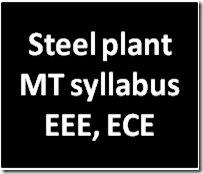Recently Visakhapatnam steel plant releases a notification for the post of management trainee,here I am giving syllabus for the written exam for the branches electronics and electrical.Written test will comprise of General Awareness, Numerical Ability, General English, Verbal and Non Verbal Reasoning and separate paper for each discipline and there is no negative marking in the test.

Aptitude:

Logical reasoning:Deductive Logic,Inductive Logic,Conceptualization,Puzzles,Venn Diagram.
Numericals:Percentages & Averages,Profit,Loss & Discount,Ratio and Proportion,Stocks and Shares
Simple Interest & Compound Interest,Time , work and distance,Permutations & Combinations.
General awareness: GK,Current Affairs,History,polity.

Technical:

Electrical: Analog and Digital Electronics,Control Systems,Electric Circuits and Fields,Electrical and Electronic Measurements,Electrical Machines,Power Electronics and Drives,Power Systems,Signals and Systems.
Electronics: Electronics Analog electronics,Computers and Microprocessors,Control Systems
Digital Electronics,Electrical and Electronic Measurements,Industrial Electronics,Network Theory
Semiconductor Devices.

BEL Probationary engineer syllabus and practice books for electronics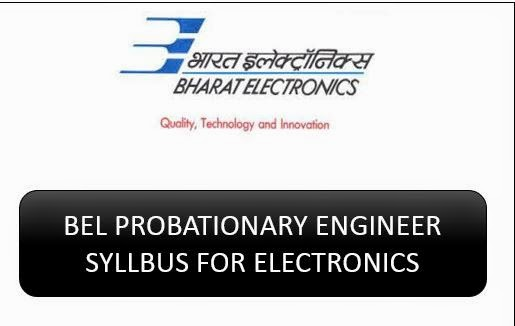Bharat electronics limited(BEL) is one of the largest electronic industry in India,every year they recruit maximum of 100 electronics engineers through GATE or a written test conducted by them and The written test will be held at Bangalore, Delhi, Mumbai, Kolkata and Guwahati centers only. So, Candidates should choose the test center nearest to their place of correspondence.

The written test is of objective type of both technical and General aptitude. In 2012 written exam they asked 150 questions with time constraint. In competitive exam time management is very important so,you should practice and prepare well to manage your exam time.

Mostly they asked basic theory questions and small problem based questions  from the engineering subjects in technical sections,the technical paper is easy for a student who preparing or prepared for GATE/IES and while coming to the aptitude it’s little bit tough,and it will take more time to solve.

see more>>>

We are giving the expected syllabus based on previous exam,there is no exact syllabus given by BEL team.
Technical section:  Follow the GATE and IES syllabus
Main Subjects: Analog electronics(concentrate more on Op-amps, Amplifiers), Basics of electronics(concentrate more on FET,MOSFET), Digital electronics,Microprocessors, Logic families, Network theory, Antennas(More important don’t leave it), Transmission lines, Signals and systems(basics of signals, Laplace transform, z-transform) , Analog and digital communications, control systems, Engineering Mathematics ( in last exam they asked questions from Differential equations,integrations and matrices), 1or 2 questions from instrumentation.

Aptitude and reasoning:  R.S.Agarwal book is sufficient.
Main Topics: Profit and loss, Simple interest and compound interest, time and work, problems on Ages, train problems, time and distance, pipes and cistern, Boats and streams so on………………

May be this year we are expecting questions for General awareness and General English also first of all you should practice technical thoroughly and then aptitude finally go for general awareness and English.

SEE MORE >> Previous Papers

Practice books:
There is no particular book for any PSU, these are all practice books which contains objective questions for practice. To success in any competitive exam practice is more important than simply studying.. All the best!!!!

For aptitude book i already wrote an article on best aptitude book you can check it here.

Practice objective question book for PSU by made easy

Objective Electrical and electronics and communications

Keywords: bel pe syllabus 2014, syllabus for bel exam electronics,bel previous papers for ece.